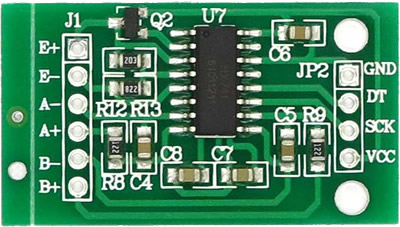upm  1.7.1 Sensor/Actuator repository for libmraa (v2.0.0)
HX711 Class Reference

API for the HX711 Analog-to-Digital Converter. More...

## Detailed Description

HX711 is a precision 24-bit analog-to-digital converter (ADC) designed for weight scales and industrial control applications to interface directly with a bridge sensor. This module was tested on the Intel(R) Galileo Gen 2 board.#include "hx711.hpp"
int
main(int argc, char** argv)
{
upm::HX711 scale(3, 2);
// 2837: value obtained via calibration
scale.setScale(2837);
scale.tare();
std::cout << scale.getUnits() << std::endl;
return 0;
}

## Public Member Functions

HX711 (int data, int sck, uint8_t gain=128)

~HX711 ()

unsigned long read ()

void setGain (uint8_t gain=128)

unsigned long readAverage (uint8_t times=10)

double getValue (uint8_t times=10)

float getUnits (uint8_t times=1)

void tare (uint8_t times=10)

void setScale (float scale=1.f)

## Constructor & Destructor Documentation

 HX711 ( int data, int sck, uint8_t gain = `128` )

HX711 constructor

Parameters
 data Defines the data pin sck Defines the clock pin gain Defines the gain factor Valid values are 128 or 64 for channel A; channel B works with a 32-gain factor only
 ~HX711 ( )

HX711 destructor

## Member Function Documentation

 unsigned long read ( )

Waits for the chip to be ready and returns a reading

Returns
 void setGain ( uint8_t gain = `128` )

Sets the gain factor; takes effect only after a call to read() channel A can be set for a 128 or 64 gain; channel B has a fixed 32-gain factor depending on the parameter; the channel is also set to either A or B

Parameters
 gain Defines the gain factor
 unsigned long readAverage ( uint8_t times = `10` )

Returns an average reading

Parameters
 times Defines how many reading to do
Returns
 double getValue ( uint8_t times = `10` )

Returns (readAverage() - OFFSET)

Parameters
 times Defines how many readings to do
Returns
Current value without the tare weight
 float getUnits ( uint8_t times = `1` )

Returns getValue() divided by SCALE

Parameters
 times Defines how many readings to do
Returns
Raw value divided by a value obtained via calibration
 void tare ( uint8_t times = `10` )

Sets the OFFSET value for the tare weight

Parameters
 times Defines how many times to read the tare value
 void setScale ( float scale = `1.f` )

Sets the SCALE value This value is used to convert the raw data to human-readable data (measurement units)

Parameters
 scale Value obtained via calibration

The documentation for this class was generated from the following files: